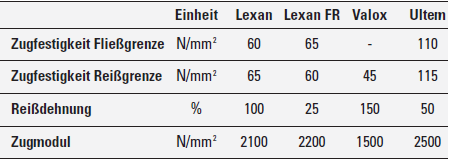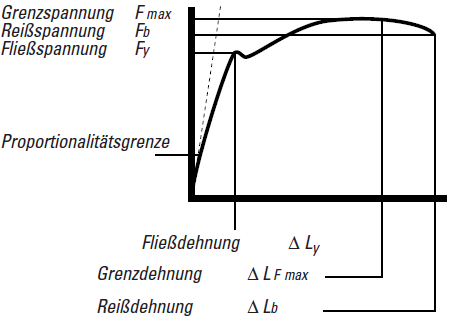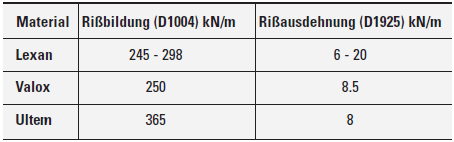# Tensile behaviour

Tensile strength, elongation and tensile modulus describe the behaviour of a film under tensile stress. Chart 8 states typical values for GE films at room temperature.

Chart 8: Tensile behaviour at room temperatureThese properties are important for the usage of adhesive tapes as well as at processing of films on rolls.

# Tension and elongation

Tension-/elongation curves illustrate the short-term load capacity. Diagram 2 shows a typical curve with a definition of the parameter.

Diagram 2: Typical tension-/elongation curveTensions can be calculated with the help of fundamental technical equations for mechanical properties. They are based on Hooke's law of elasticity, therefore the tension acts directly proportional to the elongation in the linear region. Above the proportional limit the material behaviour differs from this constant.

According to Hooke, it applies:

E= σ / ε
Whereat: σ = Tensile strength (N/mm2)
E = elasticity modulus (N/mm2)
ε = Elongation (mm/mm)

Like most thermoplasts, Lexan®, Valox® and Ultem® also show a resilient behaviour at tensile strength in the area up to the proportional limit. That means that the films elongate and when the tensile is relieved, they return in their original status. The conjoined measurement differences should be considered during printing and/or die-cutting tense films.

This behaviour can be calculated relative precise or compensate with the help of this formula:

ε = F / A x E
Whereat: ε = Elongation (mm/mm)
F = Draw (N)
A = Cross-section area of the film (mm², width x thickness)
E = Tensile modulus (N/mm²), depends on the temperature

The value “E” changes with temperature.

Under tension a corresponding change of width can be quantified with the help of the Poisson ratio. This number is 0.38 for Lexan® and Valox® films (Ultem® films 0.42). When multiplied by the elongation from the above mentioned formula, it can be calculated how much the width minimizes.

# Tear resistance

The tear resistance of a film complies with the needed strength until there are formation or distension of cracks generated. The tension is noted and the tear resistance or tear propagation strength is usually staten in Newton. It can also be phrased as strength per thickness. Maybe then it is not possible to state relevant comparisons between different materials and/or films of different thicknesses. Chart 9 mentions tear resistances, measured according to ASTM D1004 and ASTM D1925.

Chart 9: tear resistance and –propagation strength# Friction behaviour

Valox® films are an excellent surface material for numerous load-bearing applications because of their high smooth walls. Valox® also offers very low static and dynamic frictional coefficients in contact with metals. The static frictional coefficient of Valox® film is 0.39 measured according to ASTM D1894.

content-middle-right-slide

content-middle-right-bottom

Λ